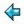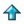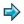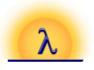### edigits.lit.m

What follows is a literate script - everything is comment except the lines beginning with >

We wish to write a program to generate the (decimal) digits of `e', as an infinite string. Fact - the value of `e', the base of natural logarithms, is given by the series

```        e = 1 + 1/1! + 1/2! + 1/3! + ...
```
where by n! we mean the factorial of n, `n! = n * (n-1)... * 2 * 1`

Now, we can choose to represent fractional numbers using a peculiar base system, in which the weight of the `i`'th digit after the point is `1/i!` (so note that the `carry factor' by which we must multiply a unit from the `i`'th digit when carrying it back to the `i-1`'th is `i`). Written to this funny base, `e' is just

```        2.1111111111............
```
so the string we require may be obtained by converting fractional part of the above numeral from the `funny base' to decimal. Thus
```>  edigits = "2." ++ convert (repeat 1)
```
The function `convert' takes for its argument a fraction in the funny base (here represented as an infinite list of numbers) and returns its value in decimal, as an infinite list of digits. The algorithm for converting a fraction from another base to decimal, is as follows:
-  multiply all digits by ten, and renormalise, using the appropriate carry factors
-  the whole number part of the result gives the first decimal digit
-  repeat the process on the fractional part of the result to generate the remaining digits.
Thus
```>  convert x = mkdigit (hd x'):convert (tl x')
>              where x' = norm 2 (0:map (10*) x)
>  mkdigit n = decode(n + code '0'), if n<10
```
It remains to define the function `norm' which does renormalisation. A naive (and almost correct) definition is
```   norm c (d:x) = d + e' div c : e' mod c : x'
where
(e':x') = norm (c+1) x
```
However, this is not a well-founded recursion, since it must search arbitrarily far to the right in the fraction being normalised before printing the first digit. If you try printing `edigits' with the above as your definition of norm, you will get "2." followed by a long silence.

We need a theorem which will limit the distance from which a carry can propagate. Fact: during the conversion of this fraction the maximum possible carry from a digit to its leftward neighbour is 9. (The proof of this, left as an exercise for the reader, is by induction on the number of times the conversion algorithm is applied.) This leads us to the following slightly more cautious definition of `norm'

```>  norm c (d:e:x) = d + e div c : e' mod c : x',  if e mod c + 9 < c
>                 = d + e' div c : e' mod c : x', otherwise
>                   where
>                   (e':x') = norm (c+1) (e:x)
```
Our solution is now complete. To see the results, enter mira with this file as the current script and say
```        edigits
```
Hit control-C (interrupt) when you have seen enough digits.